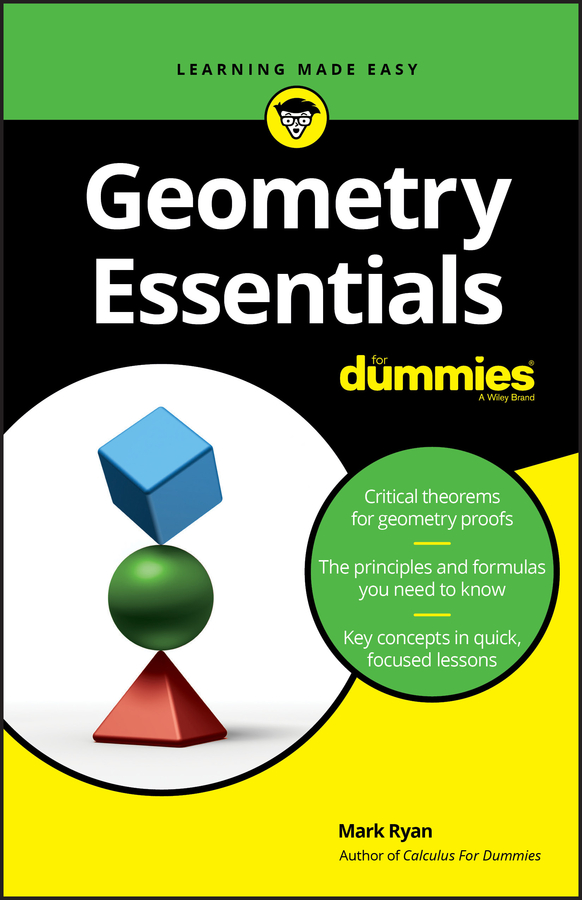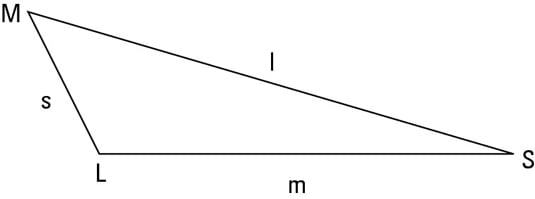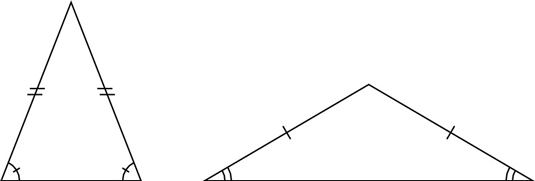##### Geometry Essentials For DummiesTriangles are classified according to the length of their sides or the measure of their angles. These classifications come in threes, just like the sides and angles themselves.

The following are triangle classifications based on sides:

• Scalene triangle: A triangle with no congruent sides

• Isosceles triangle: A triangle with at least two congruent sides

• Equilateral triangle: A triangle with three congruent sides

(For the three types of triangles based on the measure of their angles, see the article, “Identifying Triangles by Their Angles.”)

Because an equilateral triangle is also isosceles, all triangles are either scalene or isosceles. But when people call a triangle isosceles, they’re usually referring to a triangle with only two equal sides, because if the triangle had three equal sides, they’d call it equilateral. So does this classification scheme involve three types of triangles or only two? You be the judge.

## Identifying scalene trianglesIn addition to having three unequal sides, scalene triangles have three unequal angles. The shortest side is across from the smallest angle, the medium side is across from the medium angle, and — surprise, surprise — the longest side is across from the largest angle. The above figure shows an example of a scalene triangle.

The ratio of sides doesn’t equal the ratio of angles. Don’t assume that if one side of a triangle is, say, twice as long as another side that the angles opposite those sides are also in a 2 : 1 ratio. The ratio of the sides may be close to the ratio of the angles, but these ratios are never exactly equal (except when the sides are equal).

If you’re trying to figure out something about triangles — such as whether an angle bisector also bisects (cuts in half) the opposite side — you can sketch a triangle and see whether it looks true. But the triangle you sketch should be a non-right-angle, scalene triangle (as opposed to an isosceles, equilateral, or right triangle). This is because scalene triangles, by definition, lack special properties such as congruent sides or right angles.

If you sketch, say, an isosceles triangle instead, any conclusion you reach may be true only for triangles of this special type. In general, in any area of mathematics, when you want to investigate some idea, you shouldn’t make things more special than they have to be.

## Identifying isosceles trianglesAn isosceles triangle has two equal sides (or three, technically) and two equal angles (or three, technically). The equal sides are called legs, and the third side is the base. The two angles touching the base (which are congruent, or equal) are called base angles. The angle between the two legs is called the vertex angle. The above figure shows two isosceles triangles.

## Identifying equilateral triangles

An equilateral triangle has three equal sides and three equal angles (which are each 60°). Its equal angles make it equiangular as well as equilateral. You don’t often hear the expression equiangular triangle, however, because the only triangle that’s equiangular is the equilateral triangle, and everyone calls this triangle equilateral. (With quadrilaterals and other polygons, however, you need both terms, because an equiangular figure, such as a rectangle, can have sides of different lengths, and an equilateral figure, such as a rhombus, can have angles of different sizes.)